# Algebra 1 : How to find the answer to an arithmetic sequence

## Example Questions

### Example Question #2731 : Algebra Ii

Which of the following is an example of an arithmetic sequence?

Each of these sequences is an arithmetic sequence.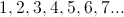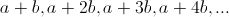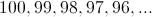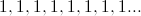Each of these sequences is an arithmetic sequence.

Explanation:

In each case, the terms increase by the same number, so all of these sequences are arithmetic.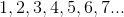Each term is the result of adding 1 to the previous term. 1 is the common difference.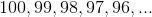Each term is the result of subtracting 1 from - or, equivalently, addingto - the previous term.is the common difference.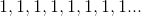The common difference is 0 in a constant sequence such as this.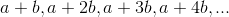Each term is the result of addingto the previous term.is the common difference.

### Example Question #12 : Arithmetic Series

Which of the following numbers completes the arithmetic sequence below?

{13, 25, __, 49}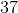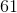Explanation:

In an arithmetic sequence the amount that the sequence grows or shrinks by on each successive term is the common difference. This is a fixed number you can get by subtracting the first term from the second.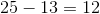So the sequence is adding 12 each time. Add 12 to 25 to get the third term.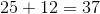So the unknown term is 37. To double check add 12 again to 37 and it should equal the fourth term, 49, which it does.